# rcs

Calculate and plot radar cross section (RCS) of platform, antenna, or array

## Syntax

``rcs(object,frequency)``
``rcs(object,frequency,azimuth,elevation)``
``rcs(___,Name,Value)``
``````[rcsval,azimuth,elevation] = rcs(object,frequency)``````
``````[rcsval,azimuth,elevation] = rcs(___,Name,Value)``````

## Description

example

````rcs(object,frequency)` plots the monostatic RCS of the platform, antenna, or array object over a specified frequency. To learn more about RCS, see What Is RCS?.```
````rcs(object,frequency,azimuth,elevation)` plots the monostatic RCS for the specified azimuth and elevation angles.```
````rcs(___,Name,Value)` plots the RCS with additional properties specified using one or more Name, Value pair arguments. This parameter can be used with any of the input arguments from the previous syntaxes.```
``````[rcsval,azimuth,elevation] = rcs(object,frequency)``` returns the RCS value of a platform, antenna, or array object at the specified frequency. `azimuth` and `elevation` are vectors over which the RCS value is calculated.```
``````[rcsval,azimuth,elevation] = rcs(___,Name,Value)``` returns the RCS value with additional properties specified using one or more Name, Value pair arguments. This parameter can be used with any of the input arguments from the previous syntaxes.```

## Examples

collapse all

Create a default helix antenna and plot the RCS at 2 GHz.

```ant = helix; rcs(ant,2e9)```Create a default linear array and plot the RCS at 75 MHz in the elevation pane.

```array = linearArray; rcs(array,75e6,0,0:1:360)```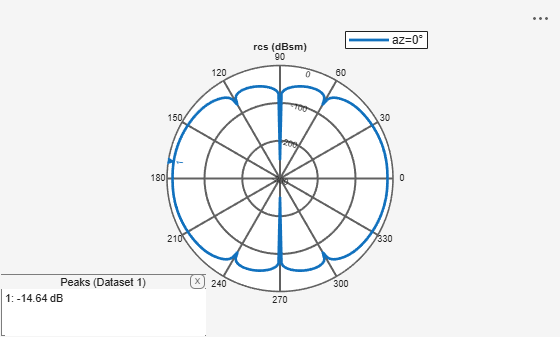Create a reflector-backed dipole and plot the RCS at 1 GHz in the elevation plane at 90 degree azimuth.

```ant = reflector; rcs(ant,1e9,90,0:1:360)```Create a tetrahedron platform from an STL file.

```p = platform; p.FileName = 'tetrahedra.stl'; p.Units = 'm'; figure show(p)```Mesh the platform with edge length of 0.1

```figure mesh(p,'MaxEdgeLength',0.1)```Sweep over the elevation with a vertically polarized E-field. Plot the RCS at 700 MHz in the azimuth plane.

```az = 0:1:360; el = 0; figure rcs(p,700e6,az,el)```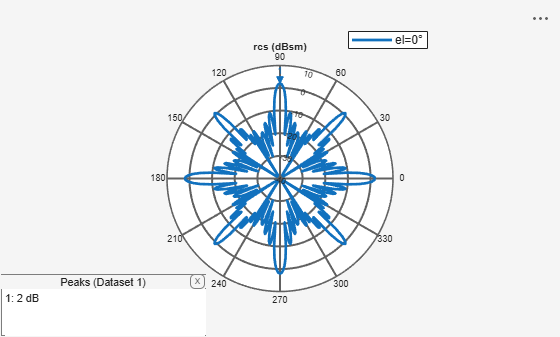Create a corner reflector-backed antenna.

```f = 2e9; c = design(reflectorCorner,750e6);```

Plot the RCS in the elevation plane.

```figure rcs(c,f,0,0:2:360)```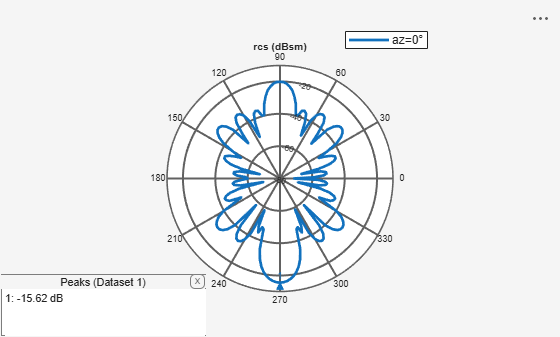Plot the RCS in the azimuth plane.

```figure rcs(c,f,0:2:360,0)```Calculate bistatic RCS for a default offset cassegrain antenna at a frequency of 14 GHz.

`S = rcs(cassegrainOffset,14e9,'TransmitAngle',[30;60],'ReceiveAngle',[30;45])`
```S = -1.2864 ```

## Input Arguments

collapse all

Platform, antenna or array element, specified as an object.

Analysis frequency, specified as a real-valued scalar in Hz.

Example: `70e6`

Data Types: `double`

Azimuth angles at which to visualize the RCS, specified as an N-element real vector in degrees.

Example: `90`

Data Types: `double`

Elevation angles at which to visualize the RCS, specified as an M-element real vector in degrees.

Example: `0:1:360`

Data Types: `double`

### Name-Value Arguments

Example: `'CoordinateSystem','polar'`

Specify optional comma-separated pairs of `Name,Value` pair arguments. `Name` is the argument name and `Value` is the corresponding value. `Name` must appear inside single quotes (`''`). You can specify several name and value pair arguments in any order as `Name1, Value1, ..., NameN, ValueN`.

Coordinate system in which to visualize the RCS, specified as the comma-separated pair consisting of `'CoordinateSystem'` and one of these values: `'polar'` or `'rectangular'`.

Example: `'CoordinateSystem','rectangular'`

Data Types: `char`

Scale at which to visualize or compute the RCS, specified as the comma-separated pair consisting of `'Scale'` and `'log'` or `'linear'`. When you choose `'log'`, the RCS is calculated and plotted in dBsm.

Example: `'Scale','linear'`

Data Types: `char`

Transmit and receive wave polarization, specified as the comma-separated pair consisting of `'Polarization'` and one of these values:

• `'HH'` – Horizontal polarized field is transmitted and received.

• `'VV'` – Vertical polarized field is transmitted and received.

• `'VH'` – Vertical polarized field is transmitted, and horizontal polarized field is received.

• `'HV'` – Horizontal polarized field is transmitted, and vertical polarized field is received.

Example: `'Polarization','VV'`

Data Types: `char`

Use GPU to perform RCS calculations, specified as the comma-separated pair consisting of `'EnableGPU'` and `0` to disable GPU or `1` to enable GPU.

Example: `'EnableGPU',1`

Data Types: `logical`

Transmit wave angle, specified as the comma-separated pair consisting of `'TransmitAngle'` and a 2-by-N real matrix representing azimuth and elevation pairs, with each element unit in degrees.

Example: `'TransmitAngle',[30;60]`

Data Types: `double`

Receive wave angle, specified as the comma-separated pair consisting of `'ReceiveAngle'` and a 2-by-M real matrix representing azimuth and elevation pairs, with each element unit in degrees.

Example: `'ReceiveAngle',[30;60]`

Data Types: `double`

Solver for RCS analysis, specified as the comma-separated pair consisting of `'Solver'` and `'PO'` (Physical Optics) or `'MoM'` (Method of Moments) or `'FMM'` (Fast Multipole Method).

Example: `'Solver'`, `'MOM'`

Data Types: `char`

Output type, specified as the comma-separated pair consisting of `'Type'` and `'Magnitude'` or `'Complex'`.

Note

Plotting rcs will error if the `'Type'` is `'Complex'`

Example: `'Type'`, `'Complex'`

Data Types: `char`

## Output Arguments

collapse all

RCS value of the platform, antenna, or array object, returned as an N-by-M real-valued array in dBsm. The size of the array is equal to the number of azimuth values (N) multiplied by the number of elevation values (M).

Azimuth angles of the calculated RCS value, returned as an N-element real-valued vector in degrees.

Elevation angles of the calculated RCS pattern, returned as an M-element real-valued vector in degrees.

collapse all

### What Is RCS?

Radar Cross Section (RCS) is the measure of scattering cross section of an object interrogated by a plane wave. The assumption of a plane wave implies that the structure is in the far field of the radiator, which is typically a part of the radar system. RCS is a function of the object's shape, the frequency of the radar, the angle of interrogation of the wave, and the object's material parameters. RCS can also be measured in logarithmic units of dBsm, which is dB relative to a 1 m2 reference area.

RCS is calculated using two typical configurations:

• Monostatic

• Bistatic

By default, the `rcs` function calculates a monostatic RCS. To calculate a bistatic RCS, restrict the `'TransmitAngle'` to 2-by-1.

Monostatic RCS

The monostatic RCS configuration is characterized by a radar system that transmits a signal and receives the backscattered signal from the object being interrogated at the same site. The source of the transmitted electromagnetic waves and the receiving system for the scattered wave are co-located.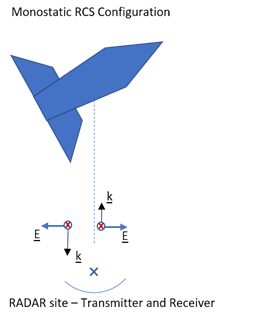Bistatic RCS

In the bistatic RCS configuration, the radar system consists of a fixed radar transmitting site and a fixed or mobile receiving site captures the backscattered waveform from the object.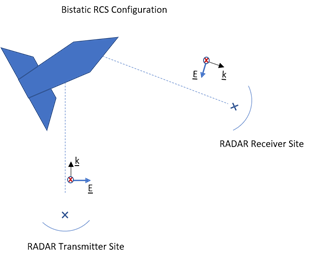### RCS Calculation

RCS is calculated in both a scalar form and a matrix form. Equations for both forms include electric (E) and magnetic (H) field quantities calculated or measured in the far field of the scattering object.

Scalar Form

In the scalar form of RCS, σ is defined as a ratio of the squared backscattered-field to the squared incident field, given by the equation:

`$\sigma =\underset{r\to \infty }{\mathrm{lim}}\text{ }\text{\hspace{0.17em}}4\pi {r}^{2}\frac{{|{E}^{\text{s}}|}^{2}}{{|{E}^{\text{i}}|}^{2}}$`

where Es and Ei represent the scattered and incident electric fields at a specific point in 3-D space.

Matrix Form

The matrix form of the RCS decomposes the incident and the scattered fields into horizontal and vertical polarizations and then computes the ratios of the various combinations between the scattered and incident fields, given by the equation:

`$\left(\begin{array}{cc}{\sigma }_{HH}& {\sigma }_{HV}\\ {\sigma }_{VH}& {\sigma }_{VV}\end{array}\right)=\underset{r\to \infty }{\mathrm{lim}}\text{ }\text{\hspace{0.17em}}4\pi {r}^{2}\left(\begin{array}{cc}\frac{{|{E}_{H}^{\text{s}}|}^{2}}{{|{E}_{H}^{\text{i}}|}^{2}}& \frac{{|{E}_{H}^{\text{s}}|}^{2}}{{|{E}_{V}^{\text{i}}|}^{2}}\\ \frac{{|{E}_{V}^{\text{s}}|}^{2}}{{|{E}_{H}^{\text{i}}|}^{2}}& \frac{{|{E}_{V}^{\text{s}}|}^{2}}{{|{E}_{V}^{\text{i}}|}^{2}}\end{array}\right)$`

where EsH and EiH represent the horizontal polarized components of the scattered and incident electric fields at a given point in 3-D space. EsV and EiV represent the vertical polarized components of the scattered and incident electric fields at a given point in 3-D space.

 Gurel, L., H. Bagrci, J. C. Castelli, A. Cheraly, F. Tardivel. "Validation Through Comparison: Measurement and Calculation of the Bistatic Radar Cross Section of a Stealth Target." Radio Science. Vol. 38, Number 3, 2003, pp.12-1 - 12-8.

 Rao, S.M., D. R. Wilton, A. W. Glisson. "Electromagnetic Scattering by Surfaces of Arbitrary Shape." IEEE Trans. Antennas and Propagation. Vol. AP-30, Number 3, 1982, pp.409-418.

 Jakobus, U., F. M. Landstorfer. "Improved PO-MM Formulation for Scattering from Three-Dimensional Perfectly Conducting Bodies of Arbitrary Shape.." IEEE Trans. Antennas and Propagation. Vol. AP-43, Number 2, 1995, pp.162-169.